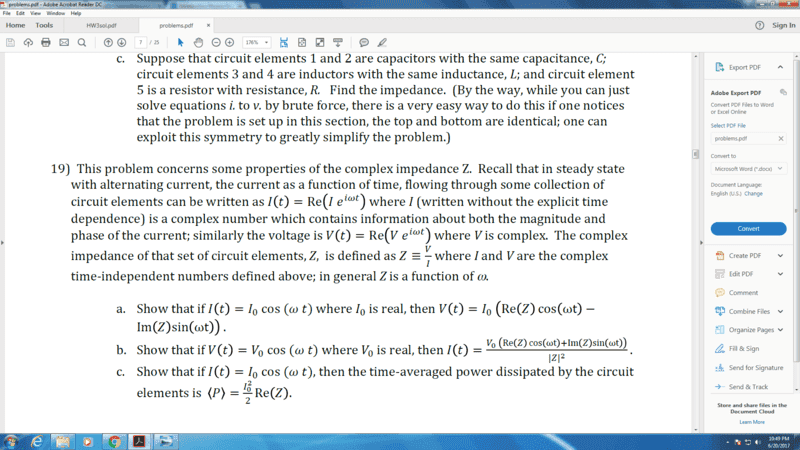# Impedance & complex currents & voltages

## Homework StatementJust problem 19C.

## Homework Equations

P=IV=Ieiwt*Veiwt. T

## The Attempt at a Solution

P = IVe2iwt=IVcos(2wt). What did I do wrong?

mjc123
Homework Helper
It asks for the time average. Integrate it over a cycle, i.e. t = 0 to 2π/ω.

ehild
Homework Helper

## Homework Statement

View attachment 205836

Just problem 19C.
=

## Homework Equations

P=IV=Ieiwt*Veiwt. T

## The Attempt at a Solution

P = IVe2iwt=IVcos(2wt). What did I do wrong?

The instantaneous power is the product of the real voltage and the real current. The complex form of power and voltage can be used for linear operations only (addition, multiplication with constant). In general, the current and voltage need not be in phase.
The complex voltage U and complex current I are related through the complex impedance Z=Ze: U=ZI. Writing in the Euler form, I=I0eiωt, U=ZI=I0Z ei(ωt+φ)=U0ei(ωt+φ).
The instantaneous power is the product or the real part of the complex current (I(t)=I0cos(ωt) with the real part of the complex voltage U(t)=U0cos(ωt+φ)), where U0=I0Z. The problem asks the average power, so you integrate the instantaneous power for a period and divide by the period T.

•Greg Bernhardt
The complex form of power and voltage can be used for linear operations only (addition, multiplication with constant).

So this means that we can't use their complex form because whatever operators we use on them are only linear? Also, is another way to understand this is that since power & voltage are real, that means we must take their real part when doing operations with them?

ehild
Homework Helper
So this means that we can't use their complex form because whatever operators we use on them are only linear? Also, is another way to understand this is that since power & voltage are real, that means we must take their real part when doing operations with them?
You can do linear operations with the complex voltages and currants, but in any other case, the real quantities should be used.